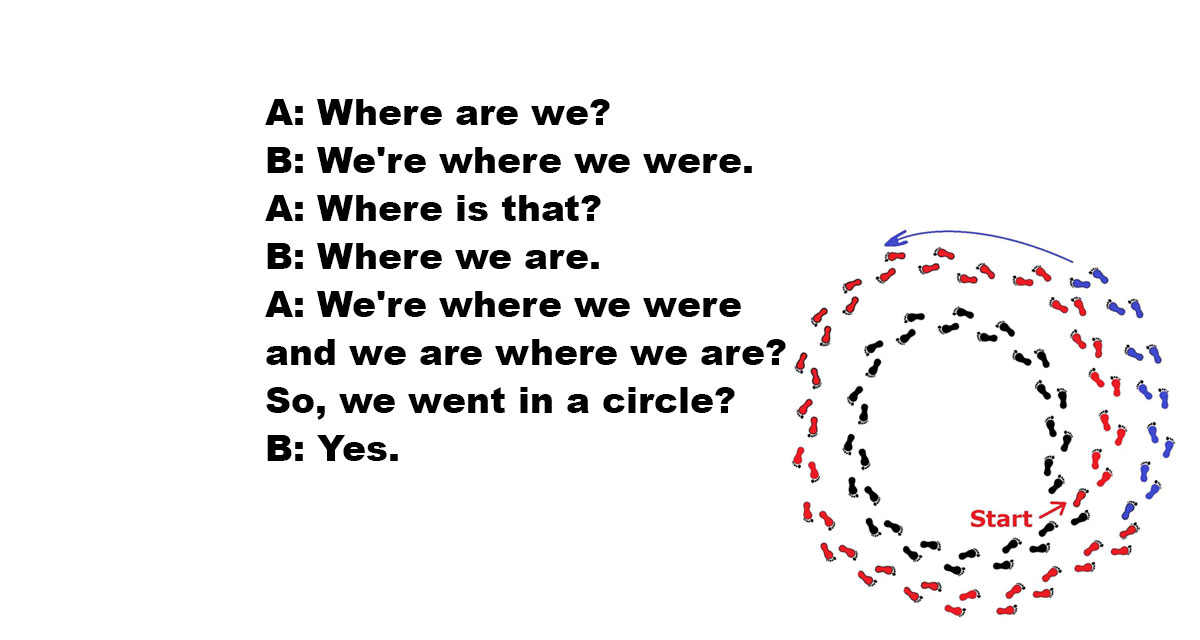## The Difference Between We’re, Where, and Were

It is common to confuse we’re, were, and where. We’re is a contraction of “we are,” where references location, and were is the plural past tense of “are.” Used in a sentence, it works like this, “we’re where we were.”

Where the sentence means, “we are, in the location, we inhabited previously”… if taken literally at least.

Or, in a short exchange:

• A: Where are we?
• B: We’re where we were.
• A: Where is that?
• B: Where we are.
• A: We’re where we were and we are where we are? So, we went in a circle?
• B: Yes.

"We’re / Where / Were" is tagged with: Philosophy of Language